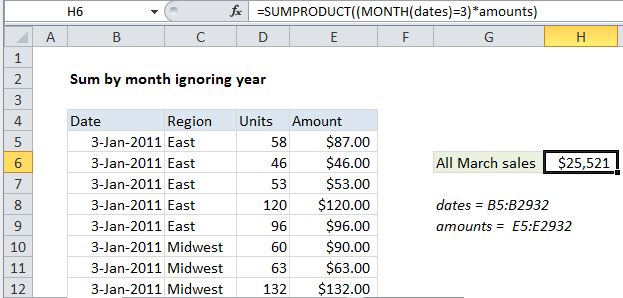## Excel Office

Excel How Tos, Tutorials, Tips & Tricks, Shortcuts

# Sum by month ignore year in Excel

This tutorial shows how to Sum by month ignore year in Excel using the example below;

## Formula

`=SUMPRODUCT((MONTH(dates)=month)*amounts)`## Explanation

To sum data by month, ignoring year, you can use a formula based on the SUMPRODUCT and MONTH functions. In the example shown, the formula in H6 is:

`=SUMPRODUCT((MONTH(dates)=3)*amounts)`

The result is a total of all sales in March, ignoring year.

### How this formula works

This data set contains over 2900 records, and the formula above uses two named ranges:

```dates = B5:B2932
amounts =  E5:E2932```

Inside the SUMPRODUCT function, the MONTH function is used to extract the month number for every date in the data set, and compare it with the number 3:

`(MONTH(dates)=3)`

If we assume a small data set listing 3 dates each in January, February, and March (in that order), the result would be an array containing nine numbers like this:

`{1;1;1;2;2;2;3;3;3}`

where each number is the “month number” for a date.  When the values are compared to 3, the result is an array like this:

`{FALSE;FALSE;FALSE;FALSE;FALSE;FALSE;TRUE;TRUE;TRUE}`

This array is then multiplied by the amount values associated with each March date. If we assume all nine amounts are equal to 100, the operation looks like this:

`{0;0;0;0;0;0;1;1;1} * {100;100;100;100;100;100;100;100;100}`

Notice the math operation changes the TRUE FALSE values into ones and zeros. After multiplication, we have a single array in SUMPRODUCT:

`=SUMPRODUCT({0;0;0;0;0;0;100;100;100})`

Note the only surviving amounts are associated with March, the rest are zero.

Worked Example:   How to determine year is a leap year in Excel

Finally, SUMPRODUCT returns the sum of all items – 300 in the abbreviated example above, and 25,521 in the screenshot with actual data.

### 2. Count by month ignoring year

To get a count by month ignoring year, you can use SUMPRODUCT like this:

`=SUMPRODUCT(--(MONTH(dates)=3))`

### Average by month ignoring year

To calculate and average by month ignoring year, you combine the two SUMPRODUCT formulas above like this:

`=SUMPRODUCT((MONTH(dates)=3)*amounts)/SUMPRODUCT(--(MONTH(dates)=3))`

## formula

`=SUMPRODUCT((MONTH(dates)=month)*amounts)`

## Explanation

To sum data by month, ignoring year, you can use a formula based on the SUMPRODUCT and MONTH functions. In the example shown, the formula in H6 is:

`=SUMPRODUCT((MONTH(dates)=3)*amounts)`

The result is a total of all sales in March, ignoring year.

### How this formula works

This data set contains over 2900 records, and the formula above uses two named ranges:

```dates = B5:B2932
amounts =  E5:E2932```

Inside the SUMPRODUCT function, the MONTH function is used to extract the month number for every date in the data set, and compare it with the number 3:

`(MONTH(dates)=3)`

If we assume a small data set listing 3 dates each in January, February, and March (in that order), the result would be an array containing nine numbers like this:

`{1;1;1;2;2;2;3;3;3}`

where each number is the “month number” for a date.  When the values are compared to 3, the result is an array like this:

`{FALSE;FALSE;FALSE;FALSE;FALSE;FALSE;TRUE;TRUE;TRUE}`

This array is then multiplied by the amount values associated with each March date. If we assume all nine amounts are equal to 100, the operation looks like this:

`{0;0;0;0;0;0;1;1;1} * {100;100;100;100;100;100;100;100;100}`

Notice the math operation changes the TRUE FALSE values into ones and zeros. After multiplication, we have a single array in SUMPRODUCT:

`=SUMPRODUCT({0;0;0;0;0;0;100;100;100})`

Note the only surviving amounts are associated with March, the rest are zero.

Worked Example:   Count cells that contain odd numbers in Excel

Finally, SUMPRODUCT returns the sum of all items – 300 in the abbreviated example above, and 25,521 in the screenshot with actual data.

### 3. Count by month ignoring year

To get a count by month ignoring year, you can use SUMPRODUCT like this:

`=SUMPRODUCT(--(MONTH(dates)=3))`

### Average by month ignoring year

To calculate and average by month ignoring year, you combine the two SUMPRODUCT formulas above like this:

`=SUMPRODUCT((MONTH(dates)=3)*amounts)/SUMPRODUCT(--(MONTH(dates)=3))`

## formula

`=SUMPRODUCT((MONTH(dates)=month)*amounts)`

## Explanation

To sum data by month, ignoring year, you can use a formula based on the SUMPRODUCT and MONTH functions. In the example shown, the formula in H6 is:

`=SUMPRODUCT((MONTH(dates)=3)*amounts)`

The result is a total of all sales in March, ignoring year.

### How this formula works

This data set contains over 2900 records, and the formula above uses two named ranges:

```dates = B5:B2932
amounts =  E5:E2932```

Inside the SUMPRODUCT function, the MONTH function is used to extract the month number for every date in the data set, and compare it with the number 3:

`(MONTH(dates)=3)`

If we assume a small data set listing 3 dates each in January, February, and March (in that order), the result would be an array containing nine numbers like this:

`{1;1;1;2;2;2;3;3;3}`

where each number is the “month number” for a date.  When the values are compared to 3, the result is an array like this:

`{FALSE;FALSE;FALSE;FALSE;FALSE;FALSE;TRUE;TRUE;TRUE}`

This array is then multiplied by the amount values associated with each March date. If we assume all nine amounts are equal to 100, the operation looks like this:

`{0;0;0;0;0;0;1;1;1} * {100;100;100;100;100;100;100;100;100}`

Notice the math operation changes the TRUE FALSE values into ones and zeros. After multiplication, we have a single array in SUMPRODUCT:

`=SUMPRODUCT({0;0;0;0;0;0;100;100;100})`

Note the only surviving amounts are associated with March, the rest are zero.

Worked Example:   Calculate Weighted Average in Excel

Finally, SUMPRODUCT returns the sum of all items – 300 in the abbreviated example above, and 25,521 in the screenshot with actual data.

### 4. Count by month ignoring year

To get a count by month ignoring year, you can use SUMPRODUCT like this:

`=SUMPRODUCT(--(MONTH(dates)=3))`

### 5. Average by month ignoring year

To calculate and average by month ignoring year, you combine the two SUMPRODUCT formulas above like this:

`=SUMPRODUCT((MONTH(dates)=3)*amounts)/SUMPRODUCT(--(MONTH(dates)=3))`